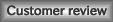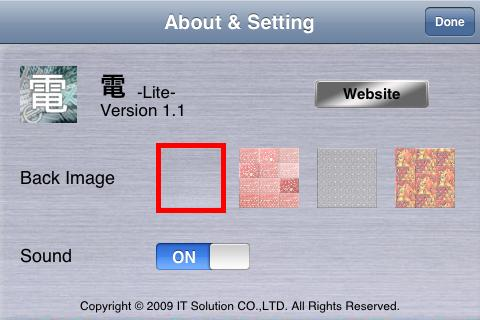## 電(E) -Lite-C language like operation calculator.

site:2009.09.24 Up

<
Information>>>
“Version1.1″ was open to the public.
-
Action of problems of number of maximum display digits when decimal is displayed.
- [+/-] Pressing operation problem action.
- Binary number, decimal number, and hexadecimal number switch operation problem action.
<<<

Various keys：
・Setting and mode changing keys
[Bin][Dec][Hex] : Change of binary mode, decimal mode and hexadecimal mode.
[8bit][16bit][32bit][64bit] : Change of bit size.
[sig][unsig] : Choice of signed or unsigned.
[i] : Change of Settings.

・Common keys of arithmetic operation and bit operation.
[Del] : Delete of 1 digit or operator.
[CLR] : Clear result.
[=] : Displays result.

・Arithmetic operator keys
[－] : Subtract operator
[x] : Multiply operator
[÷] : Divide operator
[%] : Modulo operator

・bit operator keys
[＆] : AND operator
[｜] : OR operator
[＾] : EXCLUSIVE OR operator
[~] : Tilde(NOT) operator
[<<] : Left shift operator
[>>] : right shift operator

Example of arithmetic operation:

- To calculate ( 1 + 2 ) * 3 do the following

1. select [Dec] mode
2. press 
3. press [＋]
4. press 
5. press [x]
6. press 
7. press [=]

- To calculate 7 mod 3 do the following

1. select [Dec] mode
2. press 
3. press [%]
4. press 
5. press [=]

Example of bit operation:

- To Calculate 0000 0011 AND 0000 0101 do th following

1. select [Bin] mode
2. press 
3. press [＆]
4. press 
5. press [=]

- To calculate 0A OR 07 do the following

1. select [Hex] mode
2. press [A]
3. press [｜]
4. press 
5. press [=]

- To calculate NOT 0000 0011 do the following

1. select [Bin] mode
2. press 
3. press [~]
4. press [=]

- To calculate 0000 0011 left 2bit shift do the following

1. select [Bin] mode
2. press 
3. press [<<]
4. select [Dec] mode
5. press 
6. press [=]